Question

# 6 and controller C(s), as shown in the Consider a unity-feedback control system with plant G(s)-...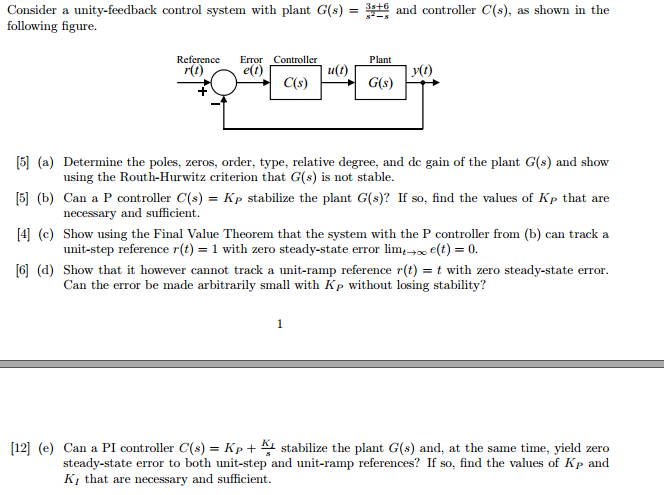6 and controller C(s), as shown in the Consider a unity-feedback control system with plant G(s)- following figure. Reference Error Controller Plant r(t) e(t) u(t) y(t) C(s) G(s)  (a) Determine the poles, zeros, order, type, relative degree, and de gain of the plant G(s) and show  (b) Can a P controller C(s)Kp stabilize the plant G(s)? If so, find the values of Kp that are  (c) Show using the Final Value Theorem that the system with the P controller from (b) can track a  (d) Show that it however cannot track a unit-ramp reference r(t)t with zero steady-state error using the Routh-Hurwitz criterion that G(s) is not stable necessary and sufficient unit-step reference r(t)1 with zero steady-state error lime(t) 0. Can the error be made arbitrarily small with Kp without losing stability? 12] (e) Can a PI controller C(s)Kpstabilize the plant G(s) and, at the same time, yield zero steady-state error to both unit-step and unit-ramp references? If so, find the values of Kp and K1 that are necessary and sufficient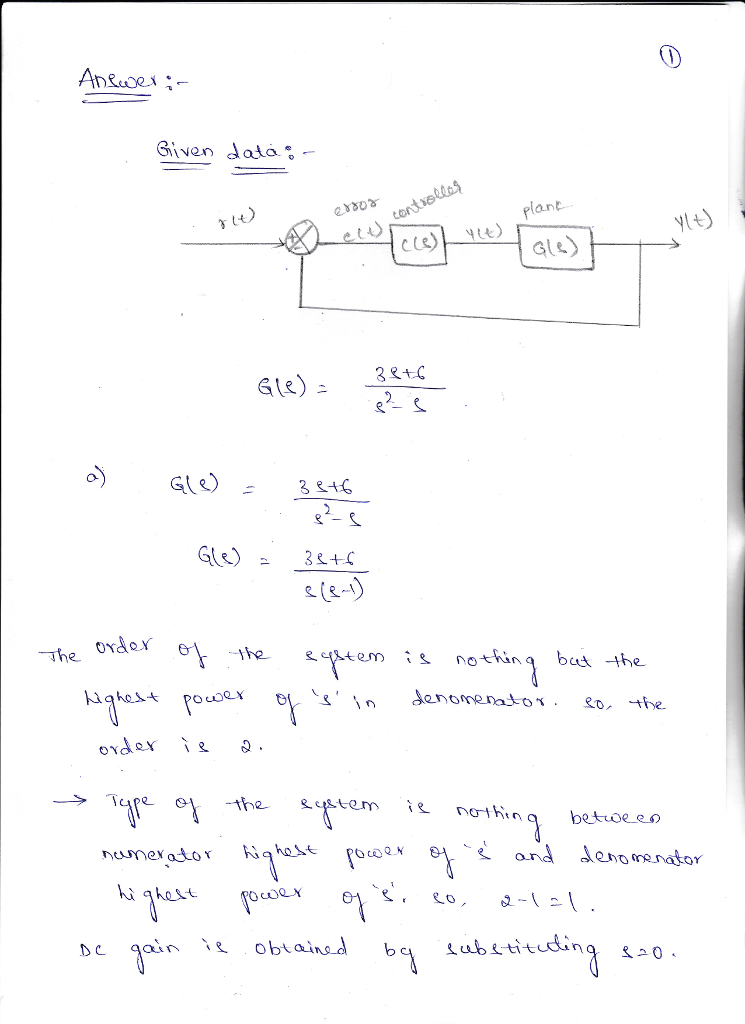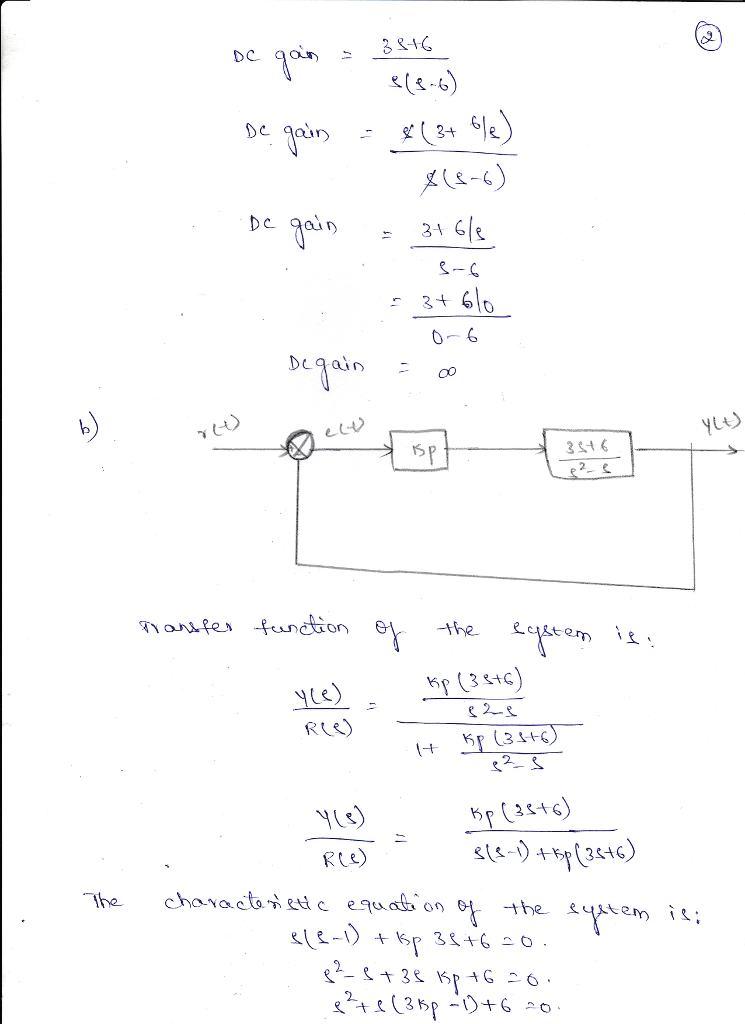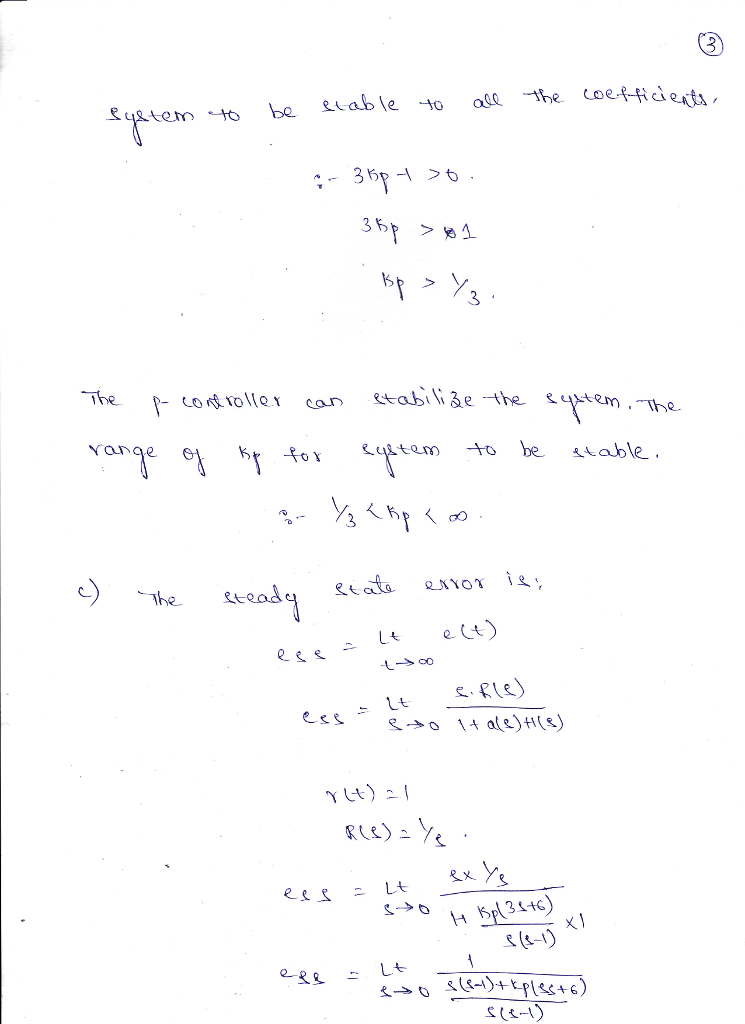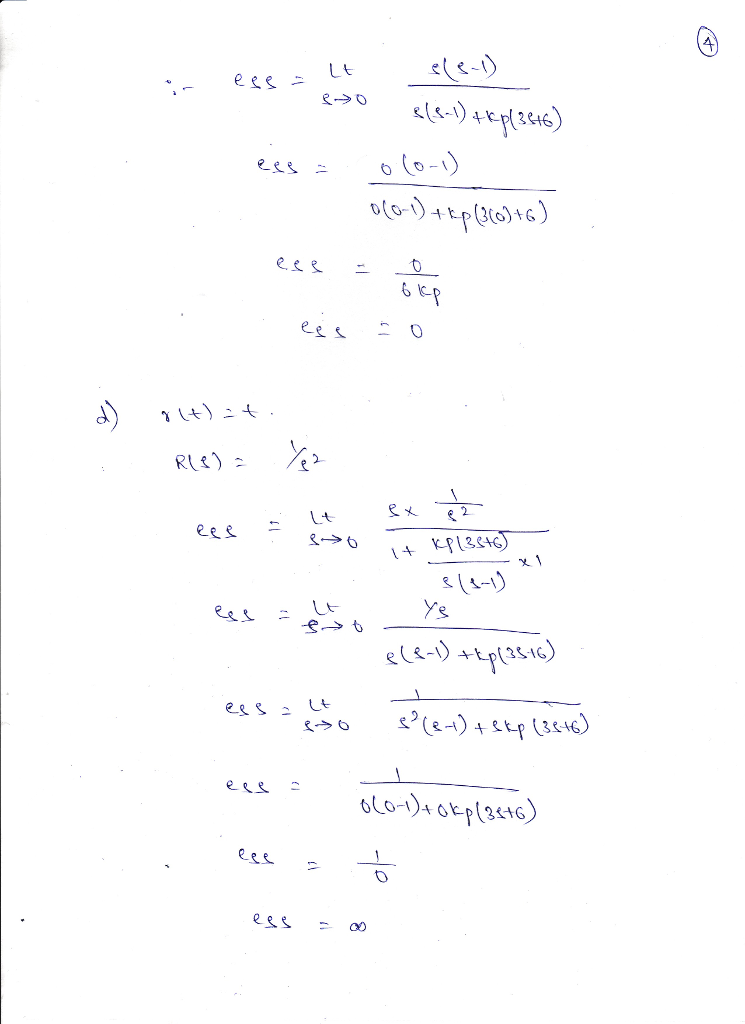#### Earn Coins

Coins can be redeemed for fabulous gifts.

Similar Homework Help Questions
• ### Consider a unity-feedback control system with a PI controller Gpr(s) and a plant G(s) in cascade. In particular, the plant transfer function is given as 2. G(s) = s+4, and the PI controller trans...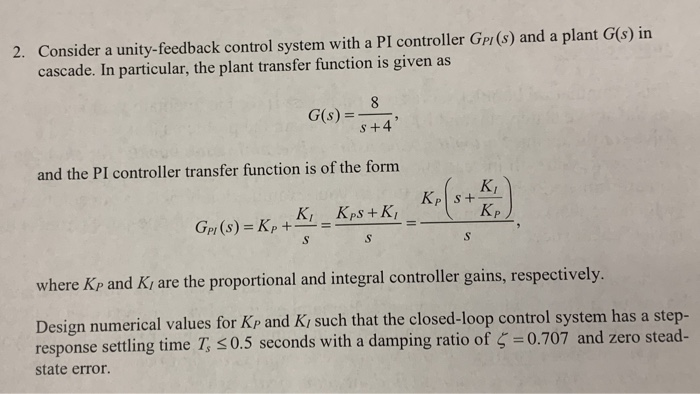Consider a unity-feedback control system with a PI controller Gpr(s) and a plant G(s) in cascade. In particular, the plant transfer function is given as 2. G(s) = s+4, and the PI controller transfer function is of the forrm KI p and Ki are the proportional and integral controller gains, respectively where K Design numerical values for Kp and Ki such that the closed-loop control system has a step- response settling time T, 0.5 seconds with a damping ratio of...

• ### 5. Consider a plant given by G()+2(s+1 in a un (s+2)(2s+1) 1n a unity feedback structure. (a) Det...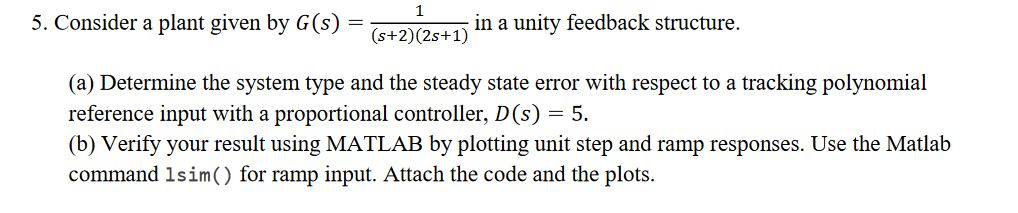5. Consider a plant given by G()+2(s+1 in a un (s+2)(2s+1) 1n a unity feedback structure. (a) Determine the system type and the steady state error with respect to a tracking polynomial reference input with a proportional controller, D(s)5. (b) Verify your result using MATLAB by plotting unit step and ramp responses. Use the Matlab command 1sim() for ramp input. Attach the code and the plots. 5. Consider a plant given by G()+2(s+1 in a un (s+2)(2s+1) 1n a unity...

• ### Controller Plant 10s+5 (s+.8)(s--1) DAG) A feedback control system is shown in Figure 4.48 (a) Determine...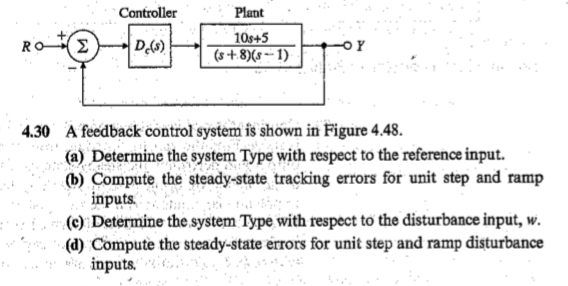Controller Plant 10s+5 (s+.8)(s--1) DAG) A feedback control system is shown in Figure 4.48 (a) Determine the system Type with respect to the reference input. (b) Compute the steady-state tracking errors for unit step and ramp inputs (e) Determine the system Type with respect to the disturbance input, w (d) Compute the steady-state errors for unit step and ramp đisturbance inputs 4.30

• ### Matlab Simulink please list all the steps Extra Credit: Simulink Disturbance Analysis D(s) Controller Plant Gp(s)...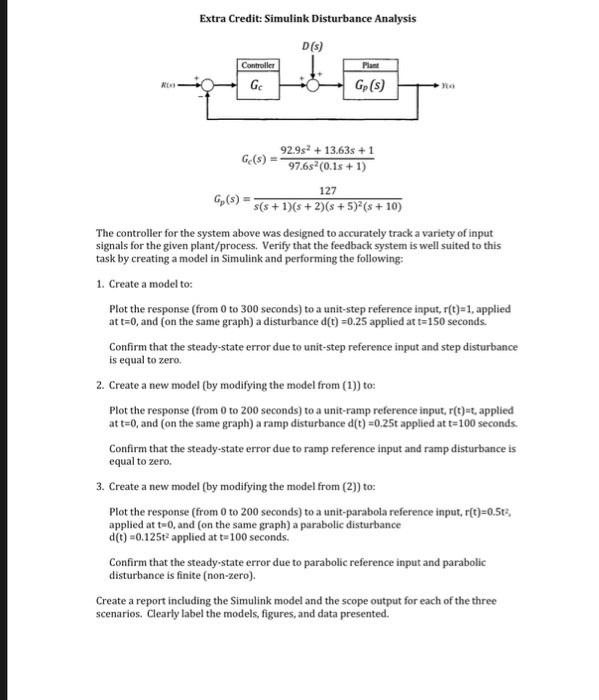Matlab Simulink please list all the steps Extra Credit: Simulink Disturbance Analysis D(s) Controller Plant Gp(s) Ge REs 92.9s213.63s 1 97.6s (0.1s + 1) 127 s(s +1)(s +2)(s 5) (s 10) Ge(s) Gp (s) The controller for the system above was designed to accurately track a variety of input signals for the given plant/process. Verify that the feedback system is well suited to this task by creating a model in Simulink and performing the following: 1. Create a model to:...

• ### answer ASAP 2. (20 points) Consider the following unity-feedback system. Suppose G(s) sis+45+6s+4) R) Ets) G(s)...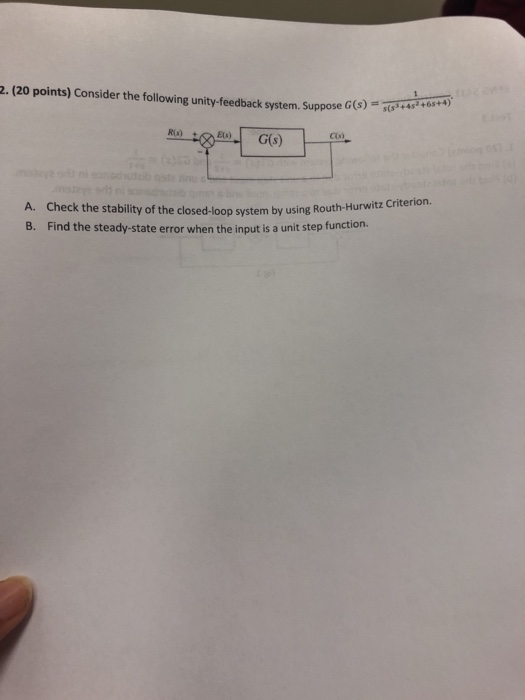answer ASAP 2. (20 points) Consider the following unity-feedback system. Suppose G(s) sis+45+6s+4) R) Ets) G(s) A. Check the stability of the closed-loop system by using Routh-Hurwitz Criterion. B. Find the steady-state error when the input is a unit step function.

• ### Consider the unity-feedback system shown below: R(s) E(s) input: r(t), output: y(t) C(s) P(s) error: e()...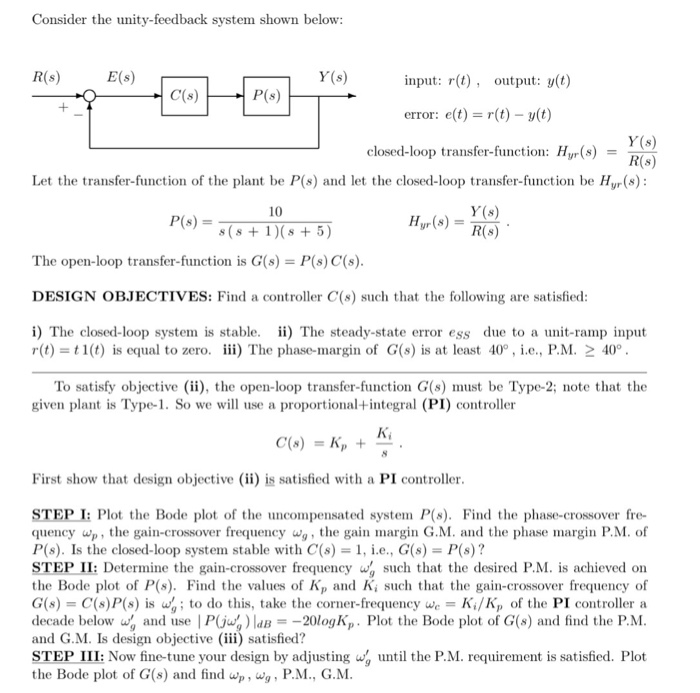Consider the unity-feedback system shown below: R(s) E(s) input: r(t), output: y(t) C(s) P(s) error: e() r(t) y(t) closed-loop transfer-function: Hyr(sD t the closed-loop transfer-function be Hyr(s) Y (s) R(s) Let the transfer-function of the plant be P(s) 10 s (s 1) (s 5) The open-loop transfer-function is G(s) P(s) C(s) DESIGN OBJECTIVES: Find a controller C(s) such that the following are satisfied i) The closed-loop system is stable. ii) The steady-state error ess due to a unit-ramp input r(t)...

• ###  Sketch the root locus for the unity feedback system whose open loop transfer function is K G(s) Draw the root lo...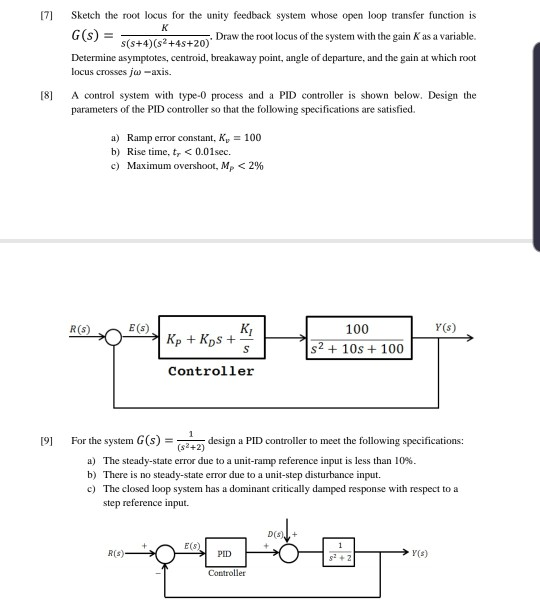Sketch the root locus for the unity feedback system whose open loop transfer function is K G(s) Draw the root locus of the system with the gain Kas a variable. s(s+4) (s2+4s+20) Determine asymptotes, centroid, breakaway point, angle of departure, and the gain at which root locus crosses ja-axis. A control system with type-0 process and a PID controller is shown below. Design the [8 parameters of the PID controller so that the following specifications are satisfied. =100 a)...

• ### 1. A system with a unity feedback has a forward transfer function of G(s) 60 G(s)...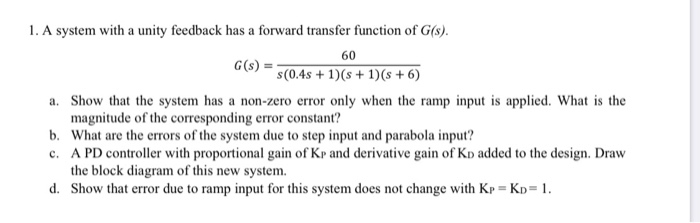1. A system with a unity feedback has a forward transfer function of G(s) 60 G(s) s(0.4s+1)(s1)(s +6) a. Show that the system has a non-zero error only when the ramp input is applied. What is the magnitude of the corresponding error constant? b. What are the errors of the system due to step input and parabola input? c. A PD controller with proportional gain of Kp and derivative gain of Kp added to the design. Draw the block diagram...

• ### 1. A feedback control system is shown in the figure below. Suppose that our design objective...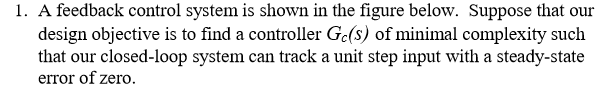1. A feedback control system is shown in the figure below. Suppose that our design objective is to find a controller Gc(S) of minimal complexity such that our closed-loop system can track a unit step input with a steady-state error of zero. (b) Now consider a more complex controller Gc(S) = [ Ko + K//s] where Ko = 2 and Ki = 20. (This is a proportional + integral (PI) controller). Plot the unit step response, and determine the steady-state...

• ### 1. [25%] Consider the closed-loop system shown where it is desired to stabilize the system with...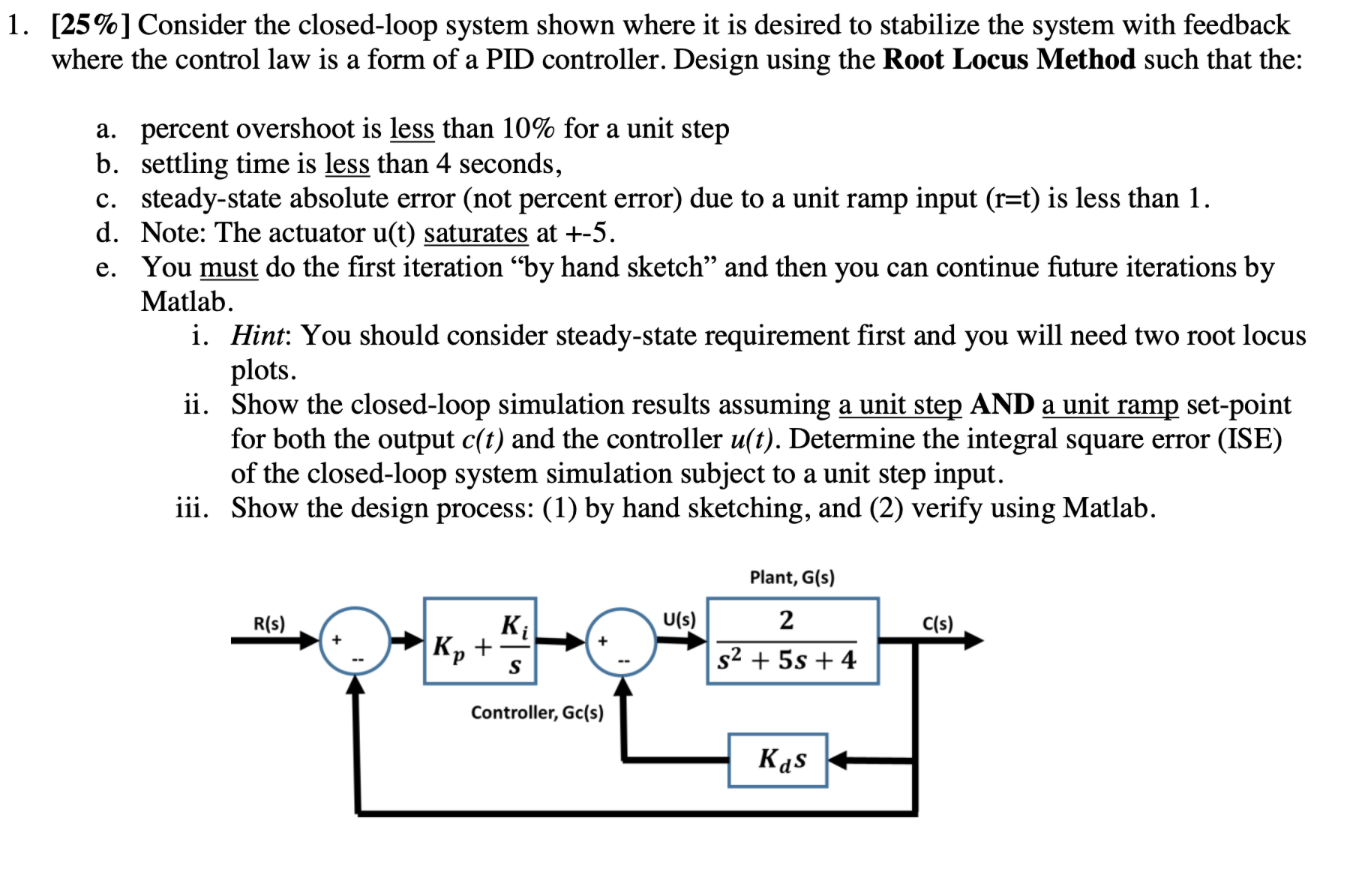1. [25%] Consider the closed-loop system shown where it is desired to stabilize the system with feedback where the control law is a form of a PID controller. Design using the Root Locus Method such that the: a. percent overshoot is less than 10% for a unit step b. settling time is less than 4 seconds, c. steady-state absolute error (not percent error) due to a unit ramp input (r=t) is less than 1. d. Note: The actuator u(t) saturates...